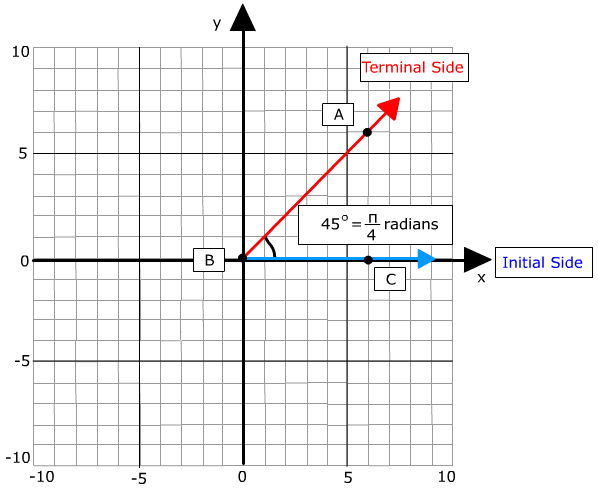# Angle Definition and Properties of Angles

An angle is a shape formed by two rays that have a common endpoint. In Trigonometry an angle is in standard position if one ray is on the positive x axis (called the initial side) and the vertex is at the origin. The space between the two rays can be measured in either degrees or radians. The terminal side can be rotated either counterclockwise or clockwise.The angle can be named in two ways: (1) if there is only one angle the vertex can be used. For example: ∠B. (2) If there are multiple angles it is best to use three points. For example: ∠ABC using the vertex as the center letter. m∠B=45° means the measure of angle B is 45 degrees.

When rotating the terminal side counterclockwise the angle measure is positive. One full rotation beginning from the initial side is 360°. However, the terminal side can rotate numerous times creating very large angles. For example 720° would be two complete revolutions.

When rotating the terminal side clockwise negative angles are created. One full rotation beginning from the initial side is -360° (negative 360°). The terminal side can be rotated numerous times creating large negative numbers. For example if the terminal side rotated 3 times the degree measure would be 1080°.

 Related Links: Math Trigonometry Introduction to the Six Trigonometric Functions (Ratios) Standard Position of an Angle - Initial Side - Terminal Side

To link to this Angle Definition and Properties of Angles page, copy the following code to your site: